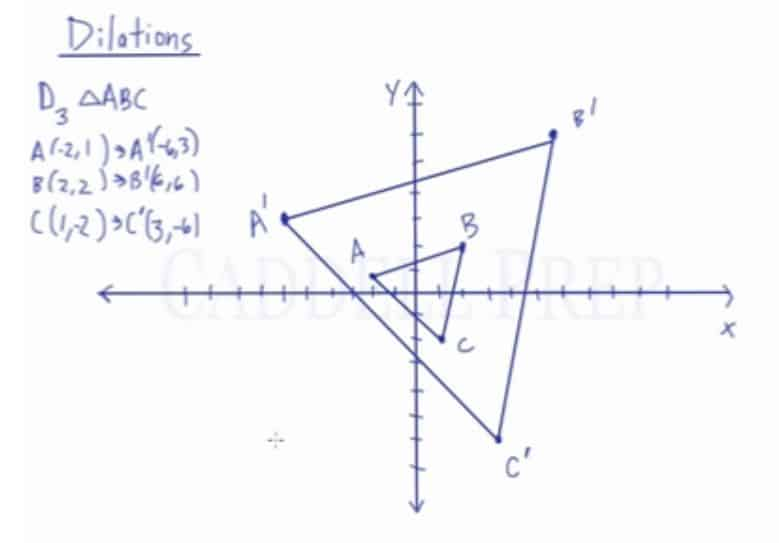In this video, you will learn how to do a dilation and graph the new image.

Dilation: Transformation of an image that is different in size but proportionate to the original figure.

Dilation Notation:
Dk(x,y)$\rightarrow$ (kx, ky) where k is the scale factor

Apply a dilation by a scale factor of 3 to triangle ABC with coordinate points of A(-2,1), B(2,2), and C(1,-2) with the center located at the origin.

Using the dilation notation and the scale factor of 3, we can easily determine the coordinate points of the image by multiplying the x-value by k and the y-value by k.

A(-2,1)$\rightarrow$ A'(-6,3)
B(2,2)$\rightarrow$ B'(6,6)
C(1,-2)$\rightarrow$ C'(3,-6)Video-Lesson Transcript

Let’s go over dilations.

Dilations are denoted by capital letter$D$ with some number$k$ before whatever it is we’re dilating.$D_{k} (x, y)$

This$k$ is our factor of dilation or also known as scaled factor.

When we do the scale factor, we just have to multiply all the coordinates by that scale factor.$D_{k} (x, y) \rightarrow (kx, ky)$

For example,$D_{4} (2, 5) \rightarrow (8, 20)$

We can see that dilation resulted in a larger coordinate. A larger$x$ value and a larger$y$ value.

If the scale factor is less than one:$D_{\dfrac{1}{2}} (2, 8) \rightarrow (1, 4)$

Here, the numbers went down.

When we dilate a shape, if$k$ is bigger than$1$ such as$4$, the shape is going to be bigger.

But if the scale factor is less than$1$ such as$\dfrac{1}{2}$, then the shape is going to be smaller.

Let’s take this triangle$ABC$ and dialte it with a scale factor of$3$.$D_3 \triangle {ABC}$

Let’s first write down our coordinates.$A (-2, 1) \rightarrow A^\prime (-6, 3)$$B (2, 2) \rightarrow B^\prime (6, 6)$$C (1, -2) \rightarrow C^\prime (3, -6)$

We just multiplied all coordinates by$3$.

Now, let’s graph this.

This is what triangle$ABC$ look like if we have a scale factor of$3$.

We just simply multiplied the$x$ and$y$ coordinates by the scale factor of$3$.

Also,$3$ is bigger than$1$ so we expect that the triangle will be bigger.

If the scale factor is less than$1$ then the triangle will be smaller than the original, depending on what the scale factor is.